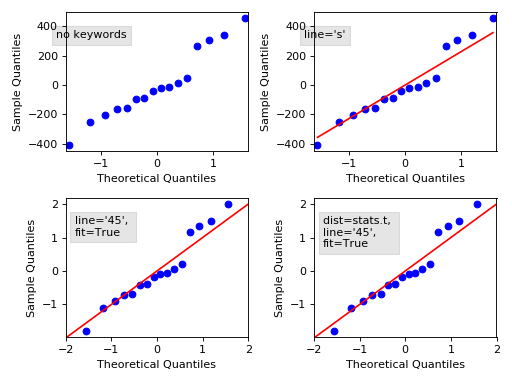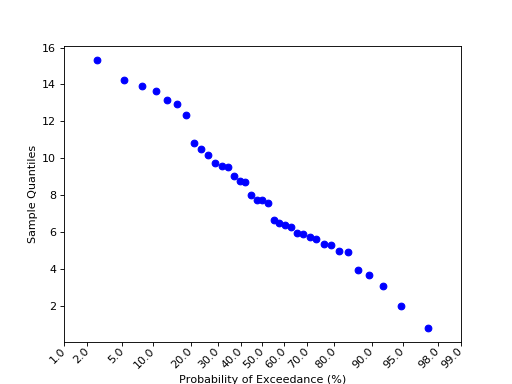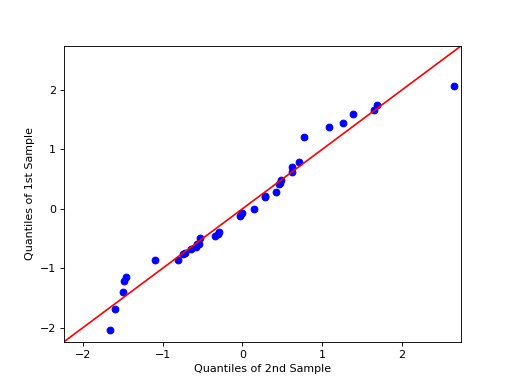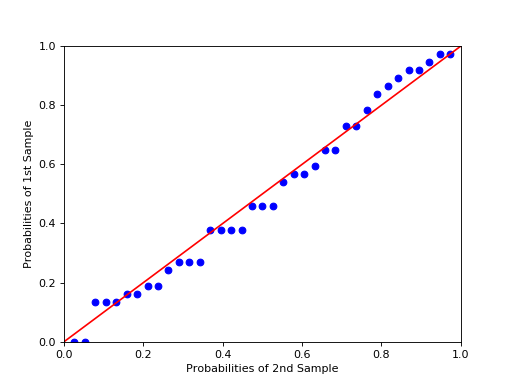statsmodels.graphics.gofplots.qqplot¶

statsmodels.graphics.gofplots.qqplot(data, dist=<scipy.stats._continuous_distns.norm_gen object>, distargs=(), a=0, loc=0, scale=1, fit=False, line=None, ax=None, **plotkwargs)[source]

Q-Q plot of the quantiles of x versus the quantiles/ppf of a distribution.

Can take arguments specifying the parameters for dist or fit them automatically. (See fit under Parameters.)

Parameters
dataarray-like

1d data array

distA scipy.stats or statsmodels distribution

Compare x against dist. The default is scipy.stats.distributions.norm (a standard normal).

distargstuple

A tuple of arguments passed to dist to specify it fully so dist.ppf may be called.

locfloat

Location parameter for dist

afloat

Offset for the plotting position of an expected order statistic, for example. The plotting positions are given by (i - a)/(nobs - 2*a + 1) for i in range(0,nobs+1)

scalefloat

Scale parameter for dist

fitboolean

If fit is false, loc, scale, and distargs are passed to the distribution. If fit is True then the parameters for dist are fit automatically using dist.fit. The quantiles are formed from the standardized data, after subtracting the fitted loc and dividing by the fitted scale.

linestr {‘45’, ‘s’, ‘r’, q’} or None

Options for the reference line to which the data is compared:

• ‘45’ - 45-degree line

• ‘s’ - standardized line, the expected order statistics are scaled by the standard deviation of the given sample and have the mean added to them

• ‘r’ - A regression line is fit

• ‘q’ - A line is fit through the quartiles.

• None - by default no reference line is added to the plot.

axMatplotlib AxesSubplot instance, optional

If given, this subplot is used to plot in instead of a new figure being created.

**plotkwargsadditional matplotlib arguments to be passed to the

plot command.

Returns
figMatplotlib figure instance

If ax is None, the created figure. Otherwise the figure to which ax is connected.

scipy.stats.probplot

Notes

Depends on matplotlib. If fit is True then the parameters are fit using the distribution’s fit() method.

Examples

>>> import statsmodels.api as sm
>>> from matplotlib import pyplot as plt
>>> mod_fit = sm.OLS(data.endog, data.exog).fit()
>>> res = mod_fit.resid # residuals
>>> fig = sm.qqplot(res)
>>> plt.show()

qqplot of the residuals against quantiles of t-distribution with 4 degrees of freedom:

>>> import scipy.stats as stats
>>> fig = sm.qqplot(res, stats.t, distargs=(4,))
>>> plt.show()

qqplot against same as above, but with mean 3 and std 10:

>>> fig = sm.qqplot(res, stats.t, distargs=(4,), loc=3, scale=10)
>>> plt.show()

Automatically determine parameters for t distribution including the loc and scale:

>>> fig = sm.qqplot(res, stats.t, fit=True, line='45')
>>> plt.show()

The following plot displays some options, follow the link to see the code.(png, hires.png, pdf)(png, hires.png, pdf)(png, hires.png, pdf)(png, hires.png, pdf)